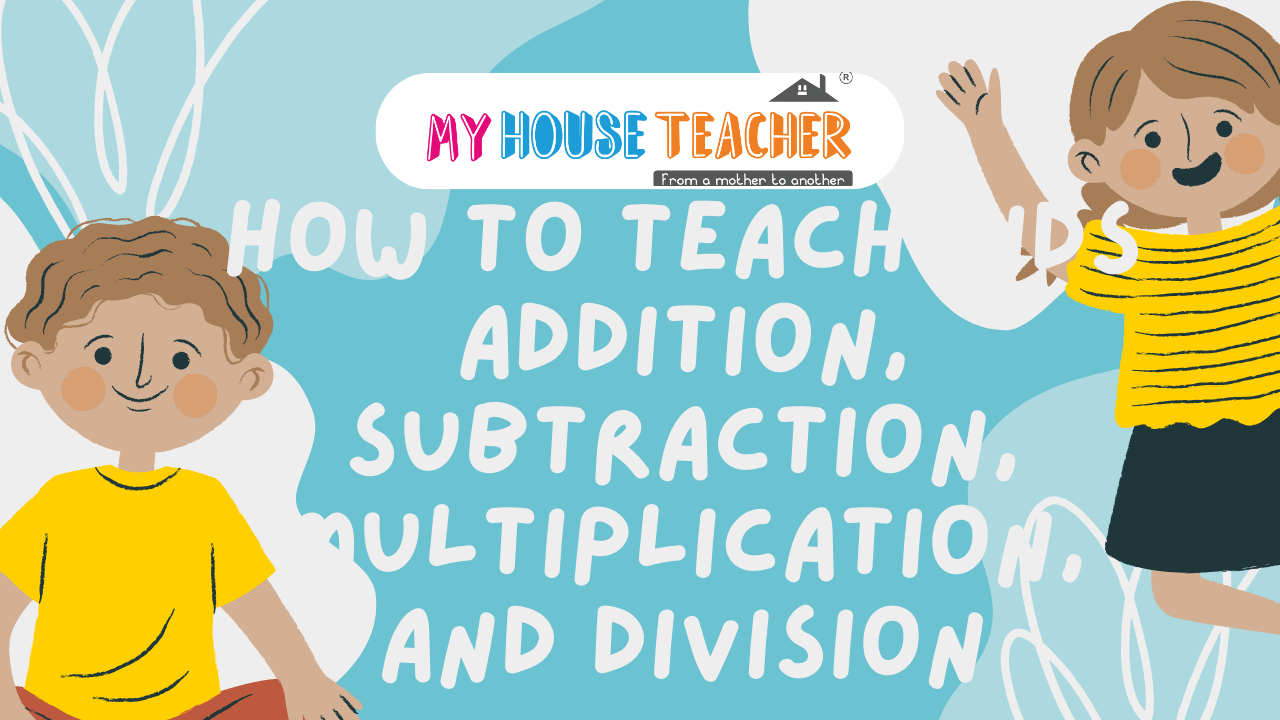# How to Teach Kids Addition, Subtraction, Multiplication, and Division

Teaching students math can be a daunting task. It requires making the kid focus on the basics and solving math problems carefully. Kids often run away from solving math questions. But teaching kids math is one of the necessary skills for their future.

Here are some of the engaging tips and strategies that you can follow to teach your kid addition, subtraction, multiplication, and division:

1. When starting with kids, one of the best approaches is to make kids focus on the basics.
2. Visual aids like number lines and abacus games help kids learn math in simple ways.
3. Kids often learn math curiously while playing games that involve math.
4. Use real-life examples to simplify the learning process for kids.
5. Take help from books, and video tutorials, to explain the concepts easily to the kids.

How to Teach Kids Addition, Subtraction, Multiplication, and Division?

### Count and Compare

Counting and Comparing is one of the basic techniques for teaching kids maths from home. It involves kids counting and math the number on the other column. It lets the kid learn to count and decide the rankings of the number.

Kids also learn to compare numbers and about greater than and less than operators. The My House teacher number comparing mat helps kids learn and compare numbers. The kits contain numbers with cute teddy bear figures. Thus, it is one of the best educational toys for kids.

Features:

1. Counting and comparing help in building the logical comparison power of a kid's mind.
2. It helps children build and analyze combinations of numbers.
3. The funny teddy bear numbers keep the kid engaged in counting and comparison.
4. Kids can improve their logical thinking capabilities.
5. The mat is of high-quality material and stays durable.

### Tracing Numbers

Tracing numbers is one of the best methods to teach kids addition and subtraction. It also helps kids learn numbers and their formation. Tracing numbers strengthens the kid's dexterity which builds a strong foundation for writing skills.

The tracing number flashcards from My house teacher are one of the best tracing mats that you can give. The set contains flashcards from 0 to 9 numbers. Kids can trace the number by following the dotted lines and then erase it for reuse.

Features:

1. The educational toy for kids helps them learn to write numbers interactively.
2. Tracing numbers helps enhance fine motor skills by following the dots of the numbers.
3. The kit contains a marker, eraser, and nine reusable flashcards for continuing the learning process.
4. Kids learn shapes and the formation of numbers in an interactive way.
5. Tracing numbers helps build confidence in kids after tracing and completing number building.

### Teaching Tables

Tables are one of the fundamental mathematical skills to teach to kids. It helps a kid develop their mental problem-solving abilities. They can perform calculations easily and quickly. Thus, it is one of the easiest ways to teach kids multiplication and improve their mental maths.

The table learning chart from My house teacher is an ideal educational toy to gift to your kid. It contains tables from 1 to 10 with reusable practice boards. It also includes a trick card for grasping mathematical tables easily.

Features:

1. It is one of the best playful ways to learn mathematical tables easily.
2. Understanding mathematical tables helps in learning other mathematical concepts like division, multiplication, etc.
3. The waterproof, tear-proof mathematical cards are for longer users.
4. It helps to enhance fluency in arithmetic concepts and problem-solving.
5. Learning tables by writing and practicing them regularly speeds up mathematical problem-solving ability.

### Magical Currency Games

Currency games are also one of the engaging activities to make kids learn numbers and mathematical concepts. There are many board games and online games that involve using fake currency notes. Kids can learn mathematical concepts like addition, division, and subtraction.

The make your kid a money genius worksheet is an ideal worksheet to make kids learn about math and money. It is an innovative board game to teach kids money and addition.

Features:

1. The game contains activity books, task sheets, practice sheets, and quiz pages.
2. You can teach your kid about saving money by spending mindfully.
3. The kids learn about subtraction by saving and spending money.
4. It helps to develop a strong foundation for numeracy skills
5. It provides them with a basic sense of economics.

### Math Puzzles

Puzzles are popular for enhancing problem-solving abilities for both kids and adults. It helps in kids' cognitive development. It enhances their problem-solving skills, number sense, and persistence. It keeps the child engaged in solving the puzzles and achieving a sense of accomplishment.

The math buzz puzzle box from My house teacher is the best educational toy for your kids. It contains several small cards with numbers and operators on them. The kid can learn various mathematical concepts like subtraction, and addition while finding the puzzle and arranging it.

Features:

1. Puzzles help improve kids' problem-solving capabilities through engaging activities.
2. It helps them improve their logical thinking abilities and learn mathematical concepts.
3. Puzzles motivate kids to solve puzzles by applying their skills and learning math.
4. It facilitates a growth mindset toward the learning process.
5.  Kids learn to identify patterns and apply mathematical concepts in solving problems.